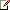# COLUMNPROPERTY (Transact-SQL)

Returns information about a column or parameter.Transact-SQL Syntax Conventions

## Syntax

``````COLUMNPROPERTY (id ,column ,property )
``````

## Arguments

• id
Is an expression that contains the identifier (ID) of the table or procedure.

• column
Is an expression that contains the name of the column or parameter.

• property
Is an expression that contains the information to be returned for id, and can be any one of the following values.

Value

Description

Value returned

AllowsNull

Allows null values.

1 = TRUE

0 = FALSE

NULL = Input is not valid.

ColumnId

Column ID value corresponding to sys.columns.column_id.

Column IDNote
When querying multiple columns, gaps may appear in the sequence of Column ID values.

FullTextTypeColumn

The TYPE COLUMN in the table that holds the document type information of the column.

ID of the full-text TYPE COLUMN for the column passed as the second parameter of this property.

IsComputed

Column is a computed column.

1 = TRUE

0 = FALSE

NULL = Input is not valid.

IsCursorType

Procedure parameter is of type CURSOR.

1 = TRUE

0 = FALSE

NULL = Input is not valid.

IsDeterministic

Column is deterministic. This property applies only to computed columns and view columns.

1 = TRUE

0 = FALSE

NULL = Input is not valid. Not a computed column or view column.

IsFulltextIndexed

Column has been registered for full-text indexing.

1 = TRUE

0 = FALSE

NULL = Input is not valid.

IsIdentity

Column uses the IDENTITY property.

1 = TRUE

0 = FALSE

NULL = Input is not valid.

IsIdNotForRepl

Column checks for the IDENTITY_INSERT setting.

1 = TRUE

0 = FALSE

NULL = Input is not valid.

IsIndexable

Column can be indexed.

1 = TRUE

0 = FALSE

NULL = Input is not valid.

IsOutParam

Procedure parameter is an output parameter.

1 = TRUE

0 = FALSE NULL = Input is not valid.

IsPrecise

Column is precise. This property applies only to deterministic columns.

1 = TRUE

0 = FALSE NULL = Input is not valid. Not a deterministic column

IsRowGuidCol

Column has the uniqueidentifier data type and is defined with the ROWGUIDCOL property.

1 = TRUE

0 = FALSE

NULL = Input is not valid.

IsSystemVerified

The determinism and precision properties of the column can be verified by the Database Engine. This property applies only to computed columns and columns of views.

1 = TRUE

0 = FALSE

NULL = Input is not valid.

IsXmlIndexable

The XML column can be used in an XML index.

1 = TRUE

0 = FALSE

NULL = Input is not valid.

Precision

Length for the data type of the column or parameter.

The length of the specified column data type

-1 = xml or large value types

NULL = Input is not valid.

Scale

Scale for the data type of the column or parameter.

The scale

NULL = Input is not valid.

SystemDataAccess

Column is derived from a function that accesses data in the system catalogs or virtual system tables of SQL Server. This property applies only to computed columns and columns of views.

1 = TRUE (Indicates read-only access.)

0 = FALSE

NULL = Input is not valid.

UserDataAccess

Column is derived from a function that accesses data in user tables, including views and temporary tables, stored in the local instance of SQL Server. This property applies only to computed columns and columns of views.

1 = TRUE (Indicates read-only access.)

0 = FALSE

NULL = Input is not valid.

UsesAnsiTrim

ANSI_PADDING was set ON when the table was first created. This property applies only to columns or parameters of type char or varchar.

1= TRUE

0= FALSE

NULL = Input is not valid.

IsSparse

Column is a sparse column. For more information, see Using Sparse Columns.

1= TRUE

0= FALSE

NULL = Input is not valid.

IsColumnSet

Column is a column set. For more information, see Using Column Sets.

1= TRUE

0= FALSE

NULL = Input is not valid.

int

## Exceptions

Returns NULL on error or if a caller does not have permission to view the object.

In SQL Server 2008, a user can only view the metadata of securables that the user owns or on which the user has been granted permission. This means that metadata-emitting, built-in functions such as COLUMNPROPERTY may return NULL if the user does not have any permission on the object. For more information, see Metadata Visibility Configuration and Troubleshooting Metadata Visibility.

## Remarks

When you check the deterministic property of a column, first test whether the column is a computed column. IsDeterministic returns NULL for noncomputed columns. Computed columns can be specified as index columns.

## Examples

The following example returns the length of the LastName column.

``````USE AdventureWorks2008R2;
GO
SELECT COLUMNPROPERTY( OBJECT_ID('Person.Person'),'LastName','PRECISION')AS 'Column Length';
GO
``````

Here is the result set.

``````Column Length
-------------
50

(1 row(s) affected)
``````